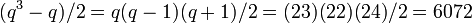# Projective special linear group:PSL(2,23)

View a complete list of particular groups (this is a very huge list!)[SHOW MORE]

## Definition

This group is defined as the projective special linear group of degree two over field:F23.

## Arithmetic functions

Want to compare and contrast arithmetic function values with other groups of the same order? Check out groups of order 6072#Arithmetic functions

### Basic arithmetic functions

Function Value Similar groups Explanation
order (number of elements, equivalently, cardinality or size of underlying set) 6072 groups with same order As$PSL(2,q), q = 23$ ($q$ odd) (see order formulas for linear groups of degree two):$(q^3 - q)/2 = q(q-1)(q + 1)/2 = (23)(22)(24)/2 = 6072$
exponent of a group 3036 groups with same order and exponent of a group | groups with same exponent of a group As$PSL(2,q)$,$q = 23$,$p = 23$ where$p$ is the characteristic:$p(q^2 - 1)/4 = 23(23^2 - 1)/4 = 3036$

### Arithmetic functions of a counting nature

Function Value Similar groups Explanation
number of conjugacy classes 14 groups with same order and number of conjugacy classes | groups with same number of conjugacy classes As$PSL(2,q), q = 23$ ($q$ odd):$(q + 5)/2 = (23 + 5)/2 = 14$
See element structure of projective special linear group of degree two over a finite field, element structure of projective special linear group:PSL(2,23)

## Group properties

Property Satisfied? Explanation Corollary properties satisfied/dissatisfied
simple group, simple non-abelian group Yes projective special linear group is simple except in finitely many cases, but this isn't one of the finite exceptions
minimal simple group Yes See classification of finite minimal simple groups
solvable group No Dissatisfies: nilpotent group, abelian group

## GAP implementation

### Short descriptions

Description Functions used
PSL(2,23) PSL
PerfectGroup(6072,1) PerfectGroup